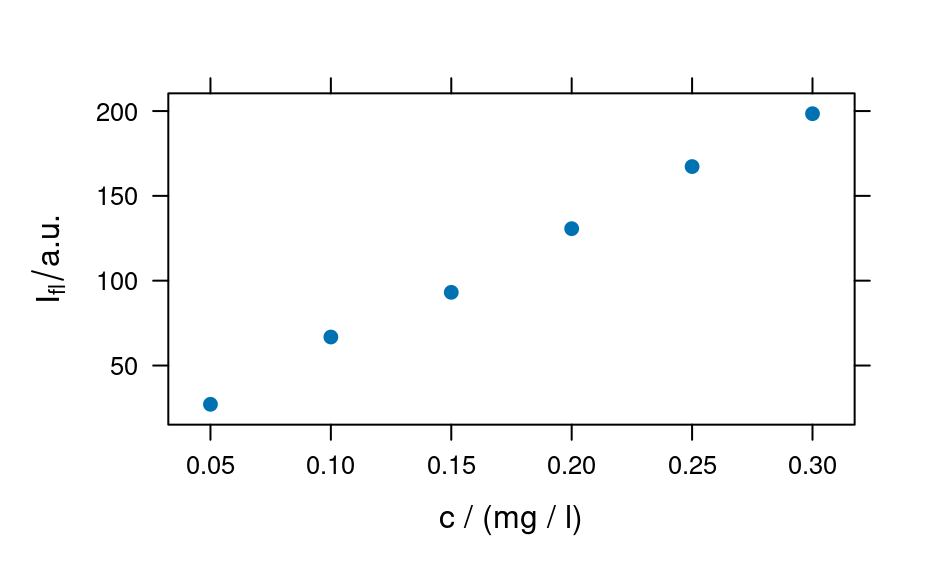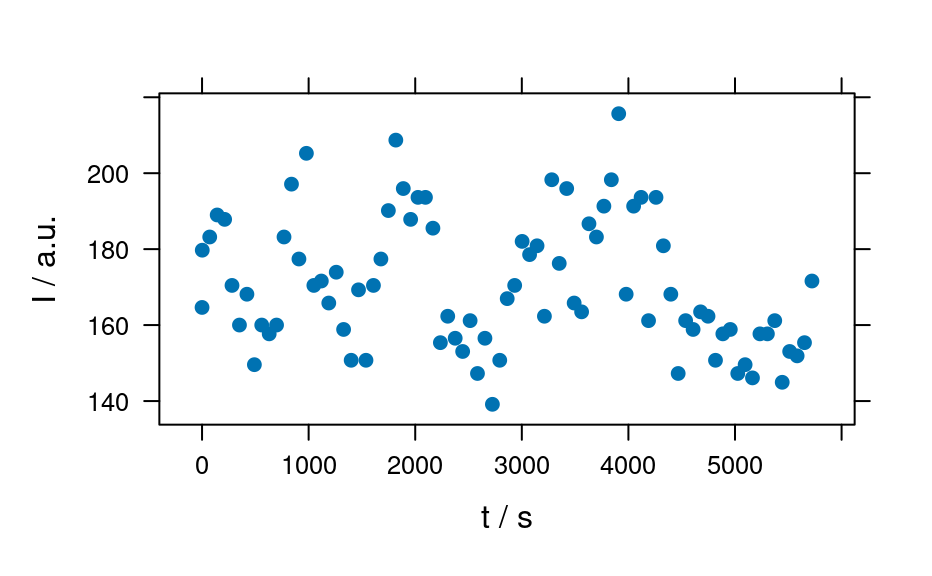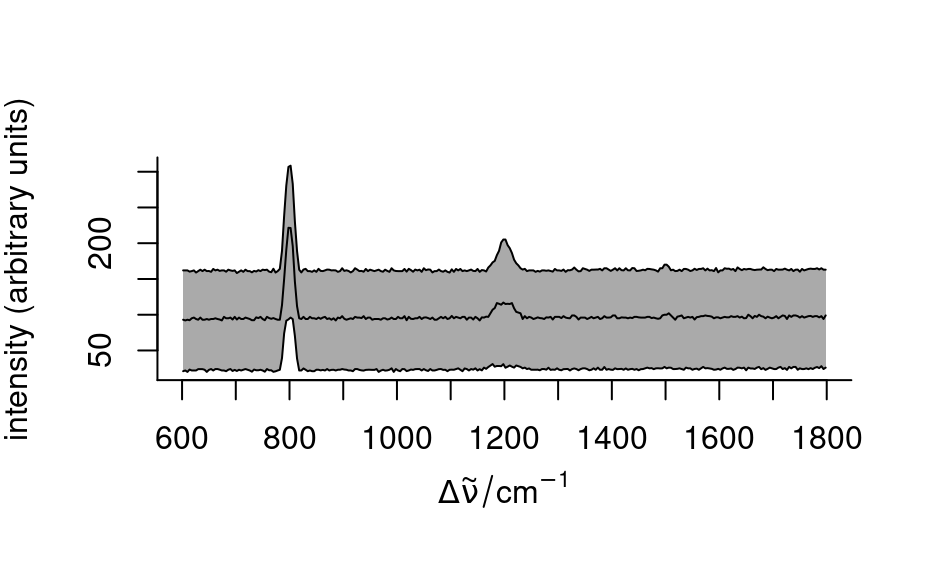The plot method for hyperSpec objects is a switchyard to plot_spc(), plot_map(), and plot_c(). The function also supplies some convenient abbreviations for frequently used plots (see 'Details').

# S4 method for hyperSpec,missing
plot(x, y, ...)

# S4 method for hyperSpec,character
plot(x, y, ...)

## Arguments

x

hyperSpec object.

y

String ("spc", "map", etc.) to select what type of plot should be produced. See section 'Details' for available values. If y is missing, plot(x) behaves like plot(x, y = "spc").

...

Arguments passed to the respective plot function

## Details

Supported values for y are:

"spc" or nothing

calls plot_spc() to produce a spectra plot.

"spcmeansd"

plots mean spectrum +/- one standard deviation

"spcprctile"

plots 16th, 50th, and 84th percentile spectra. If the distributions of the intensities at all wavelengths were normal, this would correspond to "spcmeansd". However, this is frequently not the case. Then "spcprctile" gives a better impression of the spectral data set.

"spcprctl5"

like "spcprctile", but additionally the 5th and 95th percentile spectra are plotted.

"map"

calls plot_map() to produce a map plot.

"voronoi"

calls plot_voronoi() to produce a Voronoi plot (tessellated plot, like "map" for hyperSpec objects with uneven/non-rectangular grid).

"mat"

calls plot_matrix() to produce a plot of the spectra matrix (not to be confused with graphics::matplot()).

"c"

calls plot_c() to produce a calibration (or time series, depth-profile, or the like).

"ts"

plots a time series: abbreviation for plot_c(x, use.c = "t").

"depth"

plots a depth profile: abbreviation for plot_c(x, use.c = "z").

plot_spc() for spectra plots (intensity over wavelength),

plot_map() for plotting maps, i.e. color coded summary value on two (usually spatial) dimensions.

plot_c()

graphics::plot()

C. Beleites

## Examples


plot(flu)plot(flu, "c")
#> Warning: Intensity at first wavelengh only is used.plot(laser, "ts")
#> Warning: Intensity at first wavelengh only is used.spc <- apply(faux_cell, 2, quantile, probs = 0.05)
spc <- sweep(faux_cell, 2, spc, "-")
plot(spc, "spcprctl5")plot(spc, "spcprctile")plot(spc, "spcmeansd")### Use plot_spc() as a default plot function.